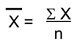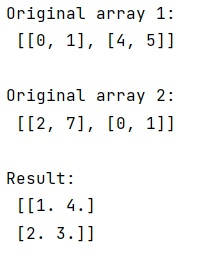# Calculate average values of two given NumPy arrays

Learn, how to calculate average values of two given NumPy arrays in Python?
Submitted by Pranit Sharma, on January 17, 2023

NumPy is an abbreviated form of Numerical Python. It is used for different types of scientific operations in python. Numpy is a vast library in python which is used for almost every kind of scientific or mathematical operation. It is itself an array which is a collection of various methods and functions for processing the arrays.

## Calculating average values of two NumPy arrays

Suppose we are given two ndarrays and we need to get the mean of the respective values between two arrays.

For this purpose, we can create a 3D array containing your 2D arrays to be averaged, then average along axis=0 using "numpy.mean() or "numpy.average().

Note: Mean is nothing but an average value of a series of a number. Mathematically, the mean can be calculated as:Here, is the mean, ∑x is the summation of all the values and n is the total number of values/elements.

Suppose we have a series of numbers from 1 to 10, then the average of this series will be:

```∑x = 1+2+3+4+5+6+7+8+9+10
∑x = 55
n = 10
x̄ = 55/10
x̄ = 5.5
```

Let us understand with the help of an example,

## Python code to calculate average values of two given NumPy arrays

```# Import numpy
import numpy as np

# Creating two numpy arrays
arr1 = [[0, 1], [4, 5]]
arr2 = [[2, 7], [0, 1]]

# Display original arrays
print("Original array 1:\n",arr1,"\n")
print("Original array 2:\n",arr2,"\n")

# Calculating result
res = np.mean( np.array([ arr1,arr2 ]), axis=0 )

# Display result
print("Result:\n",res)
```

Output: